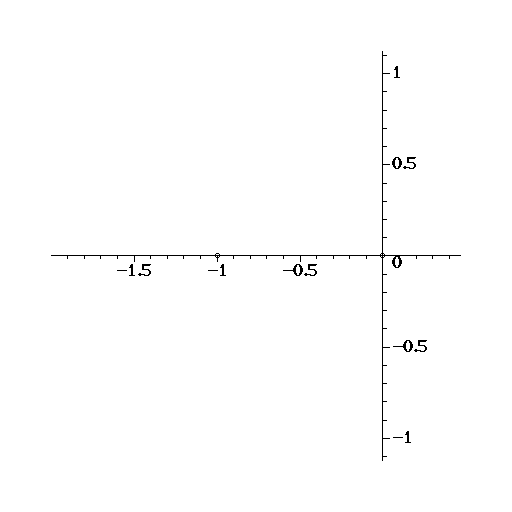### Project Goals

The Mandelbrot polynomials defined by the recurrence relation:

$p_{0}(z)=1$
$p_{k+1}(z)=z\,p_{k}^2(z)+1$

are of interest as they provide an example to test root-finding methods, in particular using generalised companion matrix pencils of Barycentric Hermite interpolants of the Mandelbrot polynomial represented as a matrix polynomial:

$P_{k+1}=\left[\begin{array}{cc} -z p_{k}(z) & 1 \\ 1 & p_{k}(z) \end{array}\right]$

The goal of this project is ultimately compute over one million roots of the Mandelbrot polynomials (i.e. compute the roots of $p_{20}(z)$). This will be achieved through the use of multiple CPU and GPU computations using sparse iterative matrix algorithms.Animation of roots of:
$\tilde{p}_{k+1}(z)=z\,p_{k}(z)^2+\epsilon$
for $\epsilon\in [0,1]$ and $k=1,\cdots,7$